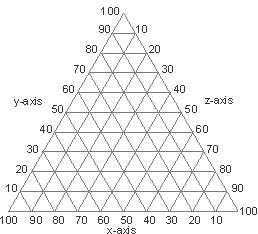soiltex

Overview

Declared in "soiltex.h"

Base class. Declared in namespace tal.

This is the class to determine the soil texture class. There are two kinds of texture class lookup: 1) Point Lookup, and 2) Range Lookup.

Point Lookup is the lookup of a texture class from a point in the texture triangle chart. You will use Point Lookup, for example, when you want to determine the USDA texture class for 35% clay and 30% sand.

Range Lookup is the lookup of texture classes from a range of points in the texture triangle chart. You will use Range Lookup, for example, when you want to determine the USDA texture classes for a range of 30-50% clay and 10-70% sand.

This class provides functionality for any soil classification scheme, provided their texture classes can be described as polygons (borders or boundaries of straight lines, not curves).

Construction, Assignment, and Destruction

soiltex()
Constructs a soiltex object. By default, the scheme name is "" (blank) and the names for the axis x, y and z are "x", "y" and "z", respectively.

soiltex(const soiltex &rhs)
Copy constructor.

soiltex &operator=(const soiltex &rhs)
Assignment operator.

virtual ~soiltex()
Destroys the soiltex object.

Classification Scheme

const std::string SchemeName() const
Returns the name of the classification scheme.

void SetSchemeName(const char *pszName)
Changes the name of the classification scheme.

void ClearScheme()
Clears the current classification scheme. All definitions for the texture classes and modified borders are cleared, the name of the classification scheme is set to "", and the names for the axis x, y and z are reverted to "x", "y" and "z", respectively.

Loads a classification scheme from a text file. How to define a soil scheme in a text file.

virtual void SaveScheme(std::fstream &f)
Saves the current classification scheme to a text file.

virtual bool IsSchemeValid() const
Checks the validity of the soil classification scheme. A valid scheme is when all the texture class polygons tile each other perfectly, do not overlap, and completely fill the area of a texture chart. If you have define your own soil scheme, it is a good idea to check its validity first before using your scheme.

Chart Axis Names

const std::string AxisX() const
Name of the x-axis in the triaxial chart.

const std::string AxisY() const
Name of the y-axis in the triaxial chart.

const std::string AxisZ() const
Name of the z-axis in the triaxial chart.

void SetAxisX(const char *pszX)
Changes the name of the x-axis in the triaxial chart.

void SetAxisY(const char *pszY)
Changes the name of the y-axis in the triaxial chart.

void SetAxisZ(const char *pszZ)
Changes the name of the z-axis in the triaxial chart.

Texture Classes

int TextureCount() const
Number of texture class definitions in the scheme.

const std::vector<TEXTURE> Texture() const
Returns a copy of all the texture class definitions in the scheme.

const TEXTURE Texture(int nIdx) const
Returns a copy of a specified texture class definition. Argument nIdx is a zero-based index of the texture class definitions.

void SetTexture(const std::vector<TEXTURE> &vtc)
Sets all texture class definitions. How to use this.

void SetTexture(int nIdx, const TEXTURE &tc)
Changes the definiton of a specified texture class. Argument nIdx is a zero-based index of the texture class definitions.

Adds a texture class definition to the scheme.

void DelTexture(int nIdx)
Deletes a specified texture class definition from the scheme. Argument nIdx is a zero-based index of the texture class definitions.

Modified Borders

int ModBorderCount() const
Number of modified border definitions in the scheme.

const std::vector<MODBOD> ModBorder() const
Returns a copy of all the modified border definitions in the scheme.

const MODBOD ModBorder(int nIdx) const
Returns a copy of a specified modified border definition. Argument nIdx is a zero-based index of the modified border definitions.

void SetModBorder(const std::vector<MODBOD> &vmb)
Sets all modified border definitions. How to use this.

void SetModBorder(int nIdx, const MODBOD &mb)
Changes the definiton of a specified modified border. Argument nIdx is a zero-based index of the modified border definitions.

Adds a modified border definition to the scheme.

void DelModBorder(int nIdx)
Deletes a specified modified border definition from the scheme. Argument nIdx is a zero-based index of the modified border definitions.

Services

const polygon RangeToPolygon(RANGE rgx, RANGE rgy, RANGE rgz=RANGE()) const
Converts a given range of values to a polygon.

Texture Class Lookup

virtual const std::vector<TEXTURE> PointLookup(double dX, double dY, double dZ=-1) const
Lookup the texture class from a point in the texture triangle chart. Arguments dX, dY and dZ are the x-, y- and z-coordinates in the triangle chart, respectively (Fig. 1). Set any of these arguments to -1 to disregard their use in the texture class lookup. For most classification schemes, dX, dY and dZ are for sand, clay and silt, respectively, but this will depend on the classification scheme.

virtual const std::vector<TEXTURE> RangeLookup(RANGE rgx, RANGE rgy, RANGE rgz=RANGE()) const
Lookup the texture classes from a range of points in the texture triangle chart. Arguments rgx, rgy and rgz are the ranges for the x-, y- and z-coordinates in the triangle chart, respectively (Fig. 1). For most classification schemes, rgx, rgy and rgz are the ranges for sand, clay and silt, respectively, but this will depend on the classification scheme.Fig. 1. Texture triangle (tri-axial) chart

TAL Home | Code Main Page | email

updated: 22-Aug-2002